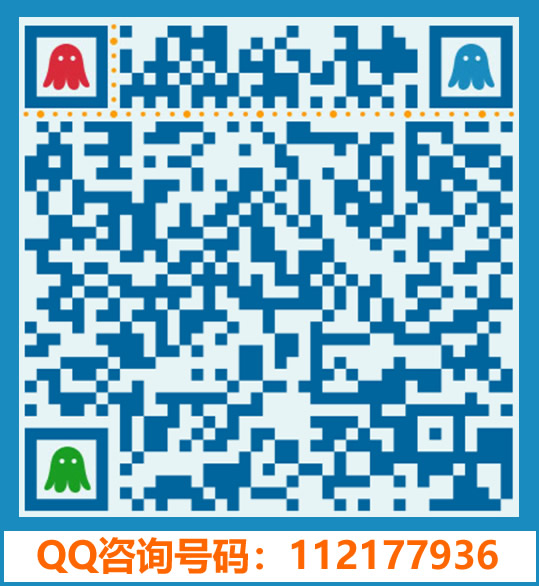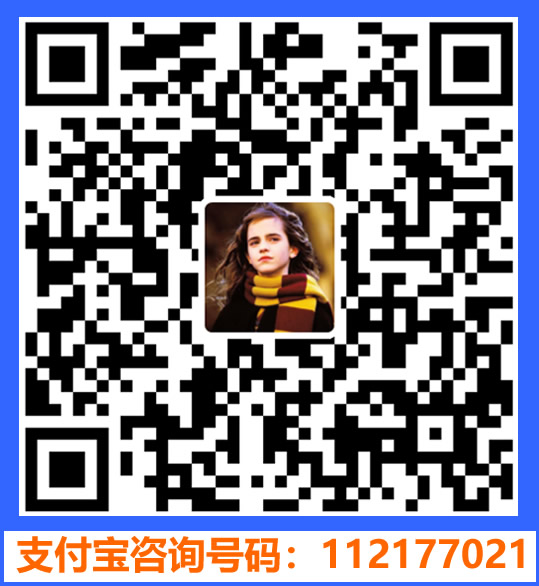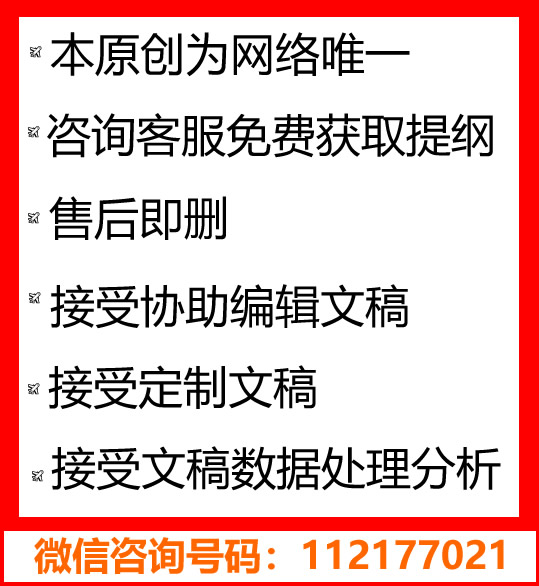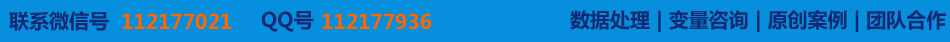# 复数法证明平面几何问题

### 〖类型:案例|7500字， 类别:原创|系数:3.4， 附件：开题报告|文献综述〗

【摘要】

复平面的建立，实现了平面几何问题与复数问题的转化，它所涉及到的数学思想是平面几何问题和代数问题的相互转化！是“数”和“型”的转化与结合的思想，在培养学生解决此问题时，不仅仅能够提供学生扩展思维的能力和解决问题的方法，还可以让学生学会数学问题的转换，深刻理解数学的作用和数学的意义，是渗透在中学的数学知识中和解题方法中的，具有普遍性和适应性的观点与认识。
复数法解决平面几何问题，主要是利用代数方法研究几何问题的一个数学方法。在解题的过程中，主要是应先把几何问题“翻译”成相应的与复数相关的问题，然后我们把解此几何问题看成是解决相应的此复数的问题，再利用我们所学的复数的有关的结论、性质来推导证明，最后我们把复数推导的结论“翻译”成所需要的结果。（通常为所要研究，推倒的几何问题的结果）这就是我们利用复数法证明平面几何问题的主要内容。

Abstract

In principle, all of plane geometry problem to solve, can be applied to analytic geometry to do it, then it can be applied to deal with complex number, because the points on the complex plane have one-to-one relationship with complex number, many plane geometry problems, especially referring to the regular pattern (eg, isosceles triangle，regular polygon, circle，rectangle, etc.) can establish the coordinate system to use complex numbers’ methods to solve.
The establishment of the complex plane achieve the transformation of the geometry and complex issues. and it involves mathematical thinking that is the conversion of the geometric problems and algebra problems! It is the transformation of “number” and “type” and combination of ideas. While training students to solve problems, it not only provide students the ability to extend thinking and methods solving problems, but also allows students to learn math problems’ conversion, and deeply understand mathematics’ role and significance. It is a universal and powerful adaptive views and understanding of penetration in the middle school mathematics knowledge and methods.
The complex method solving the Plane Geometry is a mathematical method of geometric problems using algebraic methods. In the problem-solving process, it mainly should make geometric "translate" into corresponding plural-related problems, and then we see solving geometric problems as the solution to this complex problem, re-use what we have learned plural conclusions, nature, to prove to derive .Finally, we derive conclusions of the plurality of "translation" into the needed results. (Usually the research, the results of geometric problems yea) That's the main content we use the plural methods to prove the plane geometry problems.
Key words: the plural; Plane geometry; Complex plane; Plural method

*此文为原创|获取全文在线咨询→[电脑QQ][手机QQ]【写作协助】